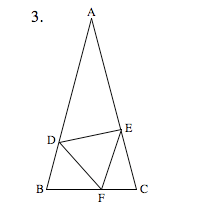## Monday, January 15, 2007

### Problems for 1-16-07

1. 9 x 10499 + 9 x 10501 = k x 10499; k = ??

2. On a certain trip, Paris covered 80% of the distance by car and the rest by bike. Her average car speed was 10 times her average bike speed. Her time spent biking was what % more than her driving time?

3.AB = AC and triangle DEF is equilateral. Points D, E and F lie on the sides of triangle ABC. The degree measure of angle A is x and the measure of angle FEC is x/2. What is the degree measure of angle DFB?

Note: Figure not drawn to scale and the answer is numerical! Show method and explain your reasoning.

Anonymous said...

1. I dunno yet.

2. Let d be the total distance, r be the bike speed, b be the time on the bike, and c be the time in the car. Distance is rate times time. So .2d = r * b and .8d = 10r * c. Then 4rb = 10rc and b = 2.5 c which means that the time spent on the bike was 2.5 times as long as the time spent in the car. Therefore, her biking time was 150% more than her driving time.

3. Since triangle ABC is isosceles, the base angles must be congruent. The vertex angle is given as x, therefore each base angle must measure (180-x)/2. Now we know two angle measures in triangle EFC, so we can subtract these from 180 to find the third. Thus, angle EFC is 90 degrees. Triangle DEF was given as equilateral, so angle DFE is 60 degrees. Finally, DFB must be 30 degrees, because it lies on a straight line with DFE and EFC.

Thanks for the good problems!

Dave Marain said...

nice job, mrc!
You'll get #1 too. My notation was klutzy. I'll spell it out: Nine times 10 raised to the 499 etc plus...

What I love is that as math teachers we're more addicted to solving these problems than our students with exceptions of course!
The real issues for me are:
1. For what grades levels (courses) are these appropriate? Only for honors classes?
2. Do they get at important concepts in math? Do they fit into typical course content? Where?
3. Is there a benefit for students from attempting challenging problems? Puzzles? Brain teasers? Even if they don't fit into the topic of the day?
4. If most are 'stuck' and begin to lose interest, what interventions should we employ? Give hints? When? How many? Give strategies? Show them a simpler but similar problem? In #1, does reducing the exponents to 1 and 3 make the problem easier or does it completely change the problem?
5. How much time does one devote to these?
6. Can they be assigned as challenges outside of class? Should our textbooks contain high level exercises such as these so that we can assign them as part of a homework assignment? If we want students to think at a higher level, shouldn't they be exposed to these on a regular basis? Hard problems seem less challenging when you're used to thinking about hard problems!
Enough questions -- enjoy the problems! I love writing these but the real challenge is getting the fonts and images to appear correctly! Am I getting better at it?
Dave

Anonymous said...

Ah, I think the answer to 1 is 909. I considered just the first 3 digits of each number, knowing that the rest would be zeros. So it's 9 + 900 = 909.

Nothing changes when this pattern takes place with 49 zeros after each number. The trick is seeing 9 * 10^501 is also 900 * 10^499.

As for your follow-on questions, I wonder a lot of the same things. I think that these kinds of questions are beneficial because they exercise thinking that's outside of the normal learning routines. In this problem, if you reduce the exponents to 3 and 1 as a "hint", I think it does change the whole thing. What's novel about this problem is that you're dealing with numbers too big to bother writing down or trying to deal with conventionally.

I don't really know how we should fit these in to our practice and into the curriculum. I would like to see students exposed to a lot of these, but it's hard to have students regularly running up against things they can't approach. It's just too easy for them to give up, but that may be a matter of being careful to pose questions at the right level of difficulty.

I know that if I were to pose the geometry question to my high school classes (mostly 10th grade) there will be 1 at most out of 80 students who will get it. Another 5 will make decent attempts. The rest will either glaze over immediately, or else scrawl nonsense. Granted, this is in a very low-performing school overall. But that also tells me that the ability to solve these kinds of puzzles is correlated with not only success on tests, but real knowledge of math.

But getting there is tough.

Anonymous said...

I used #1 in class today to soak up time from students who finished their review sheets too quickly. Since part of their work was polynomial factoring, it was natural. I helped one kid by suggesting she substitute x for 10.... The algebra can support better arithmetic.